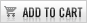New! KS2 Revision Packs

35 Booklets covering each area of the mathematics curriculum with and without answers.

Questions are grouped from previous national curriculum SATs tests and are updated annually.

Ideal for pre or post teaching, homework and revision.

Free Sample Pack
• Number - Place Value
• Measurement - Conversion
• Geometry - 2D Shapes

Complete Pack

### Complete Pack:

• All booklets listed below in the individual packs

Complete Pack

£15.00*
(Saving £2.00)Only available for the complete pack

Individual Packs

### Number Pack:

• Algebra
• Decimals
• Division
• Fractions
• Fractions, Decimals, Percentages and Equivalence
• Inverse
• Money
• Multiplication
• Negative Numbers
• Number Patterns and Sequences
• Number Properties
• Percentages
• Place Value
• Ratio
• Roman Numerals and Order of Operations
• Rounding
• Subtraction

Number Pack

£9.00*### Measurement Pack:

• Area and Perimeter
• Capacity
• Conversion
• Length
• Mass
• Scales and Temperature
• Time
• Volume

Measurement Pack

£4.00*### Geometry and Statistics Pack:

• 2D Shapes
• 3D Shapes
• Angles
• Construction and Symmetry
• Coordinates
• Transformations
• Statistics

Geometry and Statistics Pack